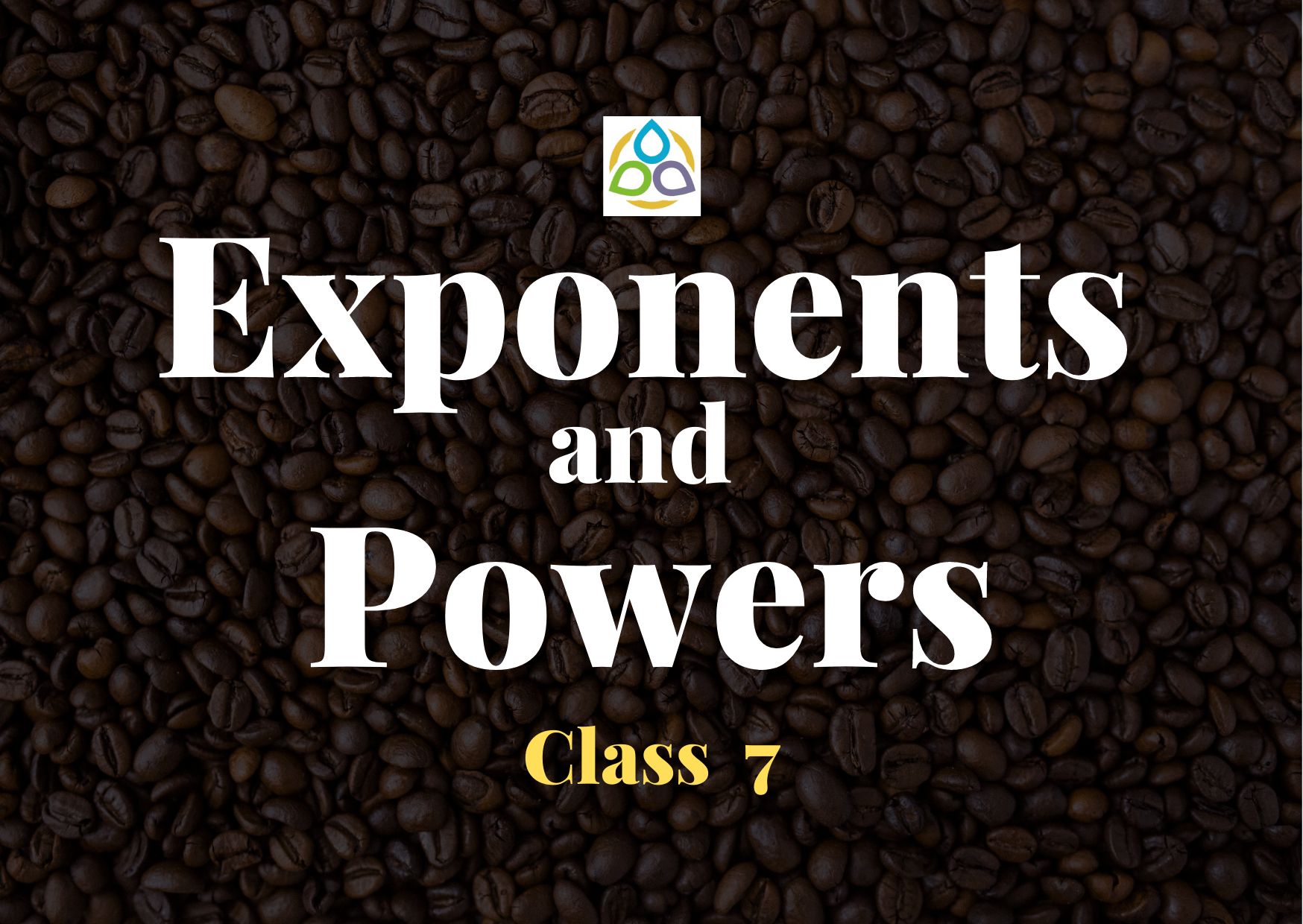# Exponents and Powers

45. Cube of (−2/3) is

• (A) −2/3
• (B) −8/27
• (C) 8/27
• (D) 2/3

44. Cube of −(5/7) is

• (A) −5/7
• (B) −125/343
• (C) 125/343
• (D) 5/7

43. Which of the following is equal to 1?

• (A) 20 + 30 + 40
• (B) 20 × 30 × 40
• (C) (30 – 20) × 40
• (D) (30 – 20) × (30 +20)

42. In standard form, the number 829030000 is written as K × 108 where K is equal to

• (A) 82903
• (B) 829.03
• (C) 82.903
• (D) 8.2903

41. In standard form, the number 72105.4 is written as 7.21054 × 10n where n is equal to

• (A) 2
• (B) 3
• (C) 4
• (D) 5

40. In standard form 72 crores is written as

• (A) 72 × 107
• (B) 72 × 108
• (C) 7.2 × 108
• (D) 7.2 × 107

39. Which of the following is not true?

• (A) 32 > 23
• (B) 43 = 26
• (C) 33 = 9
• (D) 25 > 52

38. am × an is equal to

• (A) (a2)mn
• (B) amn
• (C) am+n
• (D) amn

37. What is the value of (–8)5 × (–8)3?

• (A) 85 + 83
• (B) 88
• (C) –88
• (D) (–8)15

36. What is the simplified value of (57 ÷ 56)2?

• (A) 1
• (B) 5
• (C) 25
• (D) 125

35. [(–3)2]3 is equal to

• (A) (–3)8
• (B) (–3)6
• (C) (–3)5
• (D) (–3)23

34. For a non-zero rational number x, x8 ÷ x2 is equal to

• (A) x4
• (B) x6
• (C) x10
• (D) x16

33. x is a non-zero rational number. Product of the square of x with the cube of x is equal to the

• (A) second power of x
• (B) third power of x
• (C) fifth power of x
• (D) sixth power of x

32. For any two non-zero rational numbers x and y, x5 ÷ y5 is equal to

• (A) (x ÷ y)1
• (B) (x ÷ y)0
• (C) (x ÷ y)5
• (D) (x ÷ y)10

31. Which power of 8 is equal to 26?

• (A) 3
• (B) 2
• (C) 1
• (D) 4